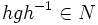# Normality satisfies image condition

This article gives the statement, and possibly proof, of a subgroup property (i.e., normal subgroup) satisfying a subgroup metaproperty (i.e., image condition)
View all subgroup metaproperty satisfactions | View all subgroup metaproperty dissatisfactions |Get help on looking up metaproperty (dis)satisfactions for subgroup properties
Get more facts about normal subgroup |Get facts that use property satisfaction of normal subgroup | Get facts that use property satisfaction of normal subgroup|Get more facts about image condition

## Statement

### Property-theoretic statement

The subgroup property of being normal satisfies the image condition: the image of a normal subgroup under any surjective homomorphism is also normal.

### Statement with symbols

Suppose$\varphi:G \to H$ is a surjective homomorphism of groups, and$N$ is a normal subgroup of$G$. Then,$\varphi(N)$ is normal in$H$.

## Generalizations

This result is part of a more general result called the fourth isomorphism theorem (also called the lattice isomorphism theorem or correspondence theorem).

## Proof

Given:$\varphi:G \to H$ is a surjective homomorphism of groups, and$N$ is a normal subgroup of$G$

To prove:$\varphi(N)$ is normal in$H$

Proof: Pick$a \in \varphi(N)$ and$b \in H$. We need to show that$bab^{-1} \in \varphi(N)$.

Since$a \in \varphi(N)$, there exists$g \in N$ such that$\varphi(g) = a$. Further, since$\varphi$ is surjective, there exists$h \in G$ such that$\varphi(h) = b$. Then:$bab^{-1} = \varphi(h)\varphi(g)\varphi(g)^{-1} = \varphi(hgh^{-1})$

(where the second step uses the fact that$\varphi$ is a homomorphism).

Now, since$N$ is normal in$G$,$hgh^{-1} \in N$, and hence$\varphi(hgh^{-1}) \in \varphi(N)$, showing that$bab^{-1} \in N$.